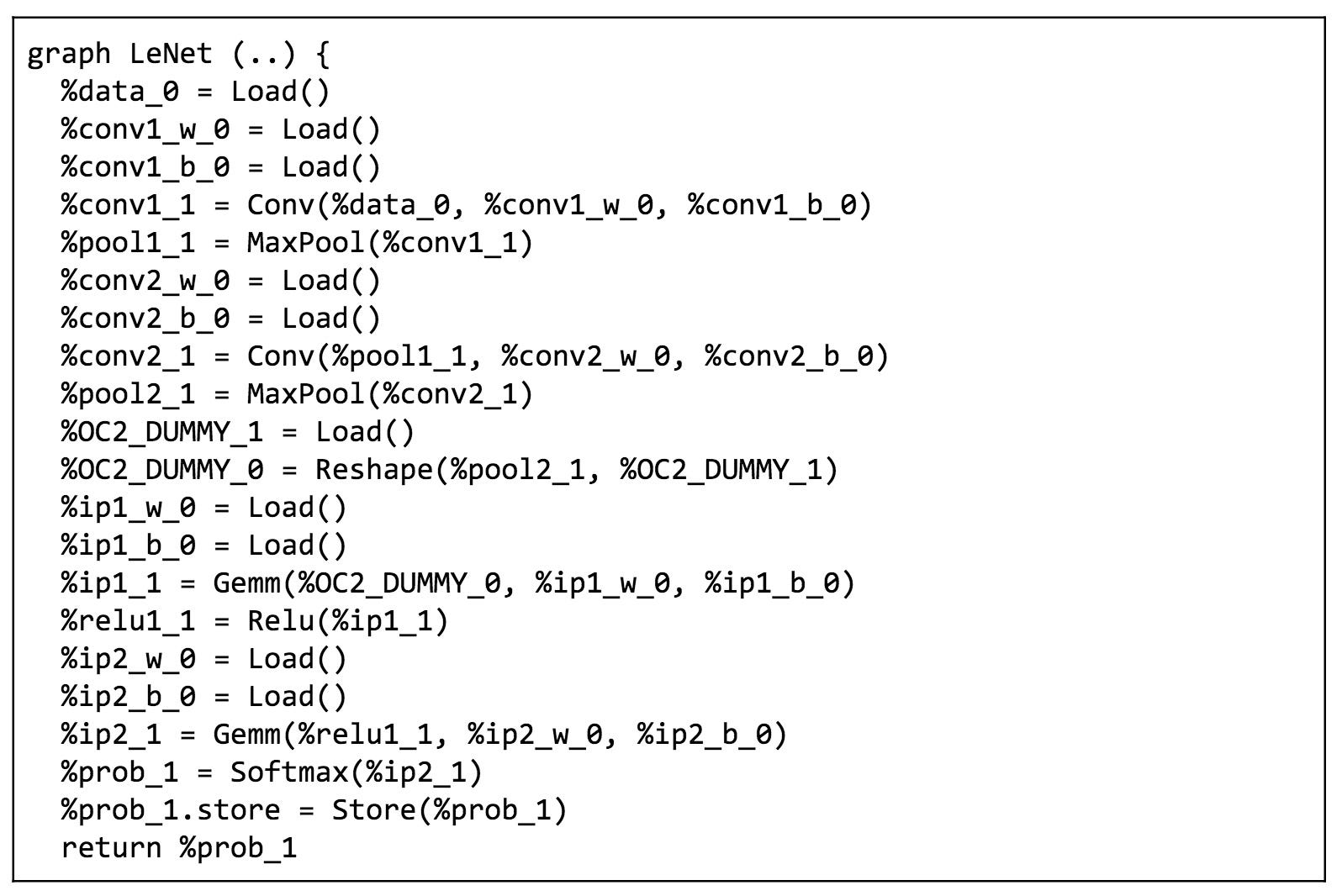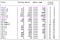# Liveness Analysis Helps Save Gigabytes of Memory Usage for AI Inference

Memory allocation is an essential step in the traditional compiler and in the neural network (NN) compiler as well. Each variable of program (or tensor of NN model) is assigned a memory space to store its value for use by later operation. In this article, we present applying to NN models a classic allocation method based on liveness analysis, and to see if this method still performs well at the NN area. The experimental results are very encouraging. On model yolo9000, for example, the memory footprint derived is only 16% of the total tensor size of the model. This is huge! Consider that the total tensor size of yolo9000 is 1.4GB. With the help of liveness analysis, only 255MB of memory is needed to store all those tensors. We save up to 1.1GB of memory!Figure 1: Memory footprint normalized to total tensor size. The smaller, the better.

Figure 1 shows more experimental results, where the value of memory footprint is normalized to total tensor size. Let’s look at the first three models yolo9000, yolov1, and VGG_ILSVRC_19_layer, the largest three models in our experiment. Around 1/3 or smaller memory footprint is required compared to the total tensor size, which means that hundreds of MB or even GB of memory is saved. The liveness based allocation helps save significant memory for huge neural network models. On average, we achieve 42% memory footprint compared to total tensor size.

Why small memory footprint does matter?

If we want to deploy models to embedded platforms, then memory footprint is a life-or-death factor. A popular platform Raspberry Pi 3 features 1GB RAM; BeagleBone Black even smaller 512MB RAM. Without appropriate memory allocation, it is however, totally impossible to deploy yolo9000 (1.4GB total tensor size) on them.

Run-time performance is also a severe issue with large memory footprint. Current CPUs or DLAs (Deep Learning Accelerators) commonly feature a hierarchical structure of memory. The smaller the memory footprint, the more chance we got to keep variables stay at the relatively small, but fast-access cache. Data movement between global system memory and local cache memory, which is time/power wasting, thus gets eliminated.

What is liveness analysis?

Liveness analysis is a classic idea to do memory allocation in traditional compilers. We say a variable (or tensor) is “alive” if its value is still needed by later operators; otherwise, no-longer used value could have its memory space released for other variables (tensors) to use. If two variables have separate liveness durations, obviously they can share same space of memory. That’s why we can shrink the memory footprint of models.

Let us consider LeNet as an example of liveness analysis. The code of Figure 2 is a kind of intermediate representation (IR) transformed from ONNX by ONNC. It describes a sequence of operations in the order of LeNet computation. Let us look at the first few operations to understand what this IR does. The first operation is to load variable %data_0 into the cache of DLA. Then the second and third mean more variables are loaded. The fourth is to do a convolution on previously loaded data %data_0, together with weight %conv1_w_0 and bias %conv1_b_0. Afterward, the result is stored as variable %conv1_1.Figure 2: IR of LeNet, transformed from ONNX by ONNC.

Now it’s time to calculate the liveness durations of tensors. For ease of discussion, assume each operator consumes exactly one unit of run time. Then, using “use-def chain” we derive the last use time of each variable. For example, %data_0 is last used by the time=3 (starting from 0) operation %conv1_1 = Conv, so its liveness duration is [0, 3]. The liveness durations of other tensors in LeNet are shown as follows at the fourth column.Figure 3: Memory allocation result for LeNet.

How to allocate memory based on liveness analysis?

With the liveness analysis result on hand, we can begin to allocate memory. The problem is formulated like this. We aim to maximize the memory sharing (equivalently to minimize the memory footprint) under the constraint that no two variables share memory if their liveness durations are overlapped. In fact, this optimization problem is NP-complete. Here, for simplicity, let’s just apply a greedy allocation. The results are shown in Figure 3. You can see variable %data_0 and %conv2_b_0 share the same memory space, while their liveness durations [0, 3] and [6, 7] respectively, are separate.

Future work

Greedy allocation is obviously not good enough. We would like to develop more advanced methods. However, our experiment shows that even with such a trivial greedy strategy, we can still derive a significant shrinking of memory footprint on some models. Liveness analysis should be an important step also in NN compilers.

The Open Neural Network Compiler (ONNC), a compiler that connects Open Neural Network Exchange Format (ONNX) to every deep learning accelerator (DLA).

## More from ONNC

The Open Neural Network Compiler (ONNC), a compiler that connects Open Neural Network Exchange Format (ONNX) to every deep learning accelerator (DLA).